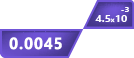# PEMDAS Calculator

Show Steps

## Other Calculators

Order of operations calculator is a basic math calculator which helps to solve math expressions. You can solve equations containing

• Subtraction
• Division
• Multiplication
• Parenthesis
• Exponent

It performs the calculations with an accurate arrangement. You can see two types of results: one is the end result and the second is a stepwise process.

## How to use Order of operations calculator?

1. Enter any non-variable expression in the input box.
2. Cross-check the expression in the display box.
3. To use special symbols click on the keyboard icon placed in the input box.
4. Click on calculate button.

## What is PEMDAS?

Pemdas meaning is:

“The order of operations in maths to solve basic math expressions for the right answer.”

Each letter in PEMDAS is the first letter of a specific math operation and the name also tells the order in which they should be solved.

## PEMDAS rules:

PEMDAS rules mean the sequence to solve a mathematical expression. The rules are simple:

1. Parentheses are the priority. If the expression has them then you will not get the correct answer if you don’t solve them first.
2. Exponents are next in line. Solve the powers after brackets.
3. Multiplication and division are to be solved after exponents.
4. And lastly, solve Addition and Subtraction.

You can choose between multiplication and division. It will not affect the answer. The same is the case with addition and subtraction. The PEMDAS calculator follows all these rules.

## When and how to use PEMDAS?

It is important to use order of operations every time you deal with an expression. Only then can you get an accurate answer. So, it is not a choice you have, you will just have to use it all the time.

As far as the question of “How to use PEMDAS?” is related, it can be solved with the help of examples.

Example:

Solve the expressions:

1. 7 + (3 x 4)2

2. (18/3)2 + ((13 + 7) x 52)

Solution:

1. The first expression is 7 + (3 x 4)2. The operations we have are parenthesis, exponent, multiplication, and addition.

Keeping the PEMDAS rules in mind, we will first solve the parentheses and there is only multiplication in brackets so it will be solved as:

= 7 + (3 x 4)2

= 7 + (12)2

Then exponents; = 7 + 122

= 7 + 144

2. The second expression, (18/3)2 + ((13 + 7) x 52), contains all PEMDAS operations except subtraction.

Following the same method, you need to see the parenthesis first. But as you can see there are three sets of brackets.

Now, we have to see if any set of brackets exists in another set. And the answer is yes. The parenthesis (13 +7) is in another set. This means it comes first.

= (18/3)2 + ((13 + 7) x 52)

= (18/3)2 + (20 x 52)

You can solve the remaining two sets side by side. As for the second parenthesis, you know that the exponents are solved before multiplication.

= (18/3)2 + (20 x 52)

= (6)2 + (20 x 25)

= 62 + 500

As done before, prioritize the power.

= 62 + 500

= 36 + 500

## FAQs

Do calculators use order of operations?

Ans: Yes, but only the scientific calculators use order of operations

Do you multiply before adding?

Ans: According to the PEMDAS rule, multiplication should be done first.

Do you multiply or divide first?

Yes, in the PEMDAS rule, multiplication and division take place before addition and subtraction